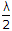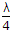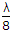# Electronics and Communication Engineering - Electromagnetic Field Theory

26.

Microwave signals transmitted towards the sky are

 A. strongly absorbed by the ionosphere B. strongly reflected by the ionosphere C. transmitted through the ionosphere D. unable to reach the ionosphere because of strong absorption in the lower atmosphere

Explanation:

No answer description available for this question. Let us discuss.

27.

Radiation intensity does not depend upon

 A. the antenna direction B. antenna width C. both (a) and (b) D. none of the above

Explanation:

No answer description available for this question. Let us discuss.

28.

Δ2V = - r/E is known as __________ equation

 A. Poisson B. Fourier C. Laplace D. Maxwell

Explanation:

No answer description available for this question. Let us discuss.

29.

A vertical earthed antenna is resonant when its physical height is equal to

 A. λ B.C.D.Explanation:

No answer description available for this question. Let us discuss.

30.

Which of the following is a vector quantity?

 A. Standing wave ratio B. Reflection coefficient C. Gain D. All of the above